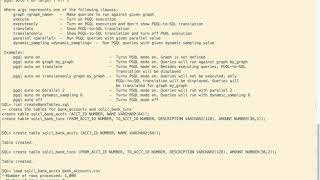# Executing Property Graph (PGQL) queries in SQLcl

Sr. Director, Product MgmtThe recent Graph Server and Client kit contains a plugin for SQLcl which enables the use of PGQL (Property Graph Query Language) for querying property graphs stored in an Oracle database.

This post is a follow-up to the blog post announcing the new kit's availability.

The description below illustrates the following:

1. Creating two tables to hold bank accounts and transactions among those accounts.
2. Creating a property graph representation of those tables.
3. Executing PGQL queries (in SQLcl) that run against the graph stored in the database.

The accompanying video is available on the Oracle Spatial and Graph YouTube channel.The first step, however, is to install the PGQL plugin. That is done as follows:

• The steps assume you have SQLcl 19.x installed and configured.
• Unzip the oracle-graph-sqlcl-plugin-20.3.0.zip into a temp directory.
• Copy the (jar) files from there into the \$SQLclHOME/lib/ext directory

Start SQLcl, after installing the plugin as above, and connect to the database (version 19c) and schema. The database can be on-premise or in the cloud.

The bank accounts CSV contains entries of the form:
ACCT_ID,NAME
1,"Account"

Similarly, the bank transactions CSV file contains entries of the form:
FROM_ACCT_ID,TO_ACCT_ID,DESCRIPTION,AMOUNT
1000,921,"transfer",1000

So let's create the tables to hold this data.

`-- drop existing tables if any`

`DROP TABLE SQLCL_BANK_ACCTS;`

`DROP TABLE SQLCL_BANK_TXNS;`

`-- create the tables `

```CREATE TABLE SQLCL_BANK_ACCTS (ACCT_ID NUMBER, NAME VARCHAR2(64)); CREATE TABLE SQLCL_BANK_TXNS (FROM_ACCT_ID NUMBER, TO_ACCT_ID NUMBER, DESCRIPTION VARCHAR2(128), AMOUNT NUMBER(38,2));```

Now load data into the tables.

`-- load accounts and transactions`

`LOAD SQLCLBANK_ACCOUNTS bank_accounts.csv;`

`LOAD SQLCL_BANK_TXNS bank_txns.csv;`

`commit;`

Next, let's create the graph from these tables. We'll use PGQL so we need to turn on the PGQL mode. That is, let SQLcl and users know that the next set of statements are in PGQL.

`-- turn on PGQL mode`

`pgql auto on;`

```-- create the property graph, i.e.  -- specify which tables become vertices and which columns of those tables become properties of those vertices -- specify which tables contain edges (i.e. links between vertices) and which columns become edge properties```

```create property graph sqlcl_bank_graph vertex tables (     SQLCL_BANK_ACCTS     Key (ACCT_ID)     Label ACCOUNT     Properties (ACCT_ID) ) edge tables (     SQLCL_BANK_TXNS     key(ROWID)     Source key (FROM_ACCT_ID) references SQLCL_BANK_ACCTS     Destination key (TO_ACCT_ID) references SQLCL_BANK_ACCTS     Label TRANSFER     Properties (FROM_ACCT_ID, TO_ACCT_ID, AMOUNT) );```

`commit;`

Graph creation usually takes 30 seconds or so for this dataset, though it depends on the database configuration.

Now enter some PGQL queries to explore this graph.

```-- Sample PGQL queries. () denotes a vertex, [] an edge, -> a directed edge, MATCH specifies a graph pattern  -- first set the default graph to sqlcl_bank_graph pgql auto on graph sqlcl_bank_graph -- count number of vertices and edges select count(v) match (v); count(v) ---------------------------------------------------------------- 1000```

```select count(e) match ()-[e]->(); count(e) ---------------------------------------------------------------- 5001```

```-- get vertex and edge Labels select distinct label(v) match (v); label(v) ---------------------------------------------------------------- ACCOUNT```

```select distinct label(e) match ()-[e]->(); label(e) ---------------------------------------------------------------- TRANSFER```

```-- check if there are circular payment chains of length 3 or 4. / / is a variable length path. :TRANSFER = edge label select /* last hop is explicitly specified */ count(*) match (v)-/:TRANSFER{2}/->(m)-[:TRANSFER]->(v); count(*) ---------------------------------------------------------------- 131```

`set sqlformat ansiconsole`

```select /* last hop is explicitly specified */ count(*) match (v)-/:TRANSFER{3}/->(m)-[:TRANSFER]->(v); count(*) ---------------------------------------------------------------- 530```

```-- get the top 5 accounts with the most number of incoming transfers select v.ACCT_ID, count(*) as NumDeposits match (m)-[e]->(v) group by v.ACCT_ID order by NumDeposits desc limit 5;    v.ACCT_ID    NumDeposits ____________ ______________ 387          39 934          39 135          36 534          32 380          31```

```-- Insert a new vertex and some edges INSERT VERTEX v1 LABELS ( ACCOUNT ) PROPERTIES ( v1.ACCT_ID = 1001), VERTEX v2 LABELS ( ACCOUNT ) PROPERTIES ( v2. ACCT_ID = 1002), VERTEX v3 LABELS ( ACCOUNT ) PROPERTIES ( v3. ACCT_ID = 1003), EDGE e1 BETWEEN v1 AND v2 LABELS ( TRANSFER ) PROPERTIES ( e1.AMOUNT = 101 ), EDGE e2 BETWEEN v2 AND v3 LABELS ( TRANSFER ) PROPERTIES ( e2.AMOUNT = 1099 ) ;```

`5 rows modified`

```-- Query the newly inserted data select s.ACCT_ID, e.AMOUNT, d.ACCT_ID MATCH (s)-[e]->(d) WHERE s.ACCT_ID=1002 or s.ACCT_ID=1001;    s.ACCT_ID    e.AMOUNT    d.ACCT_ID ____________ ___________ ____________ 1002         1099        1003 1001         101         1002```

```-- delete an edge DELETE e FROM MATCH (s)-[e]->(n) WHERE s.ACCT_ID=1002 AND n.ACCT_ID=1003; 1 row modified```

`-- rerun earlier query on newly inserted vertices and edges`
```select s.ACCT_ID, e.AMOUNT, d.ACCT_ID MATCH (s)-[e]->(d) WHERE s.ACCT_ID=1002 or s.ACCT_ID=1001;    s.ACCT_ID    e.AMOUNT    d.ACCT_ID ____________ ___________ ____________ 1001         101         1002```

And that is how you can use PGQL in SQLcl to create and query a graph stored in an Oracle database.

The corresponding product documentation contains more details.

The SQLcl plugin is described here.

PGQL is described here and the formal specification is available here.

#### Join the discussion

• Jim Dickson Tuesday, March 23, 2021
I am looking for latest workshop for Graph.
Want to run on 21c ADB (Always Free tenancy)

Ideally want PGQL to run from with SQL client (sqlplus, sql developer, sqlcl).

Ideally want to visualize/interact with results in APEX.

Jim
• Jim Steiner Friday, April 9, 2021
Jim,

The best place to find these is the Oracle LiveLabs at:
https://apexapps.oracle.com/pls/apex/f?p=133:1

Then search for "Graph". You will find a number of workshops of this type.

I would suggest:
Analyze, Query, and Visualize Graphs in Oracle Database

and

Getting Started with Graph Studio

Jim